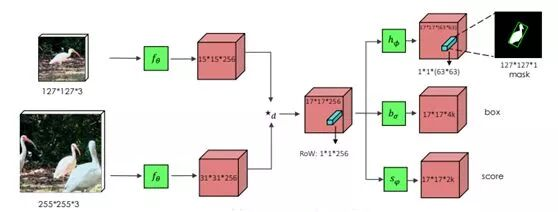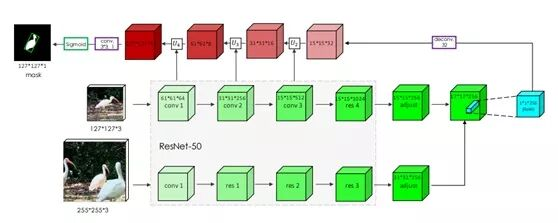# Siamese Network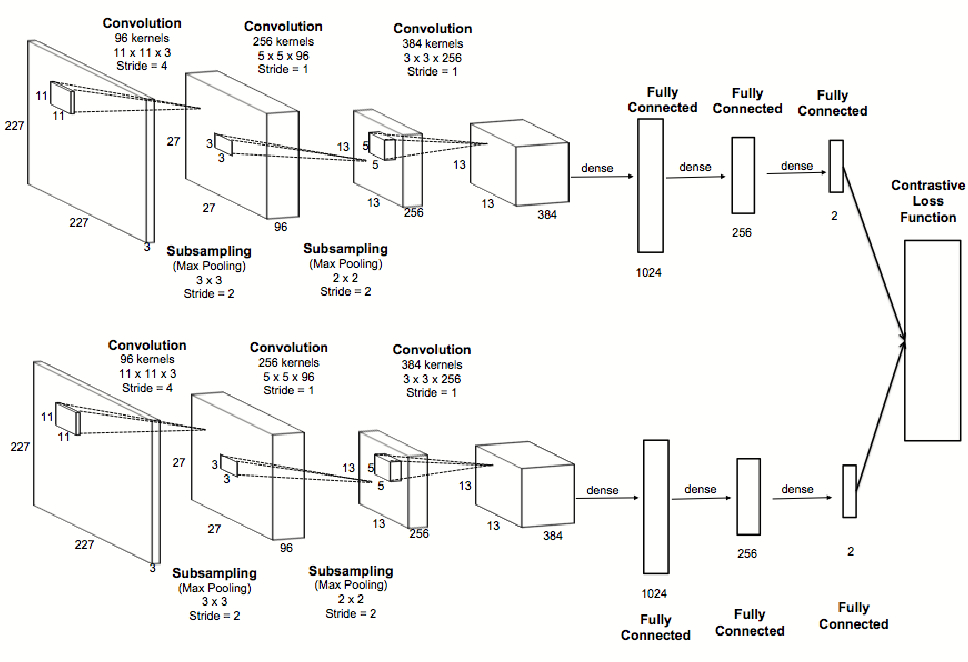Siamese network is an artificial neural network that use the same weights while working in tandem on two different input vectors to compute comparable output vectors. If the weights are not shared, it is sometimes referred as Pseudo Siamese network. For example, one input is text, the other input is image, we may need different architecture for two branches.

To compare the similarity of two inputs, contrastive loss is mostly used: $$\ell=\sum{yd+(1-y)\max{(\epsilon-d, 0)}}$$ where d is the distance function for response of two inputs, ϵ is the margin and y is the label: y=0 means two inputs are similar, vice versa.

For distance function, many choices are available, e.g., Euclidean distance, cosine distance.

We will introduce some representative work in Saimese network in the following sections.

# SiameseFC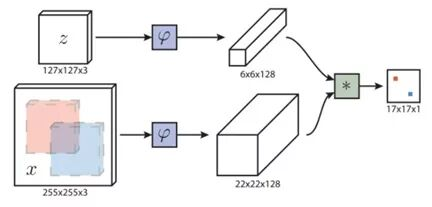SiameseFC has been proposed for visual object tracking, where z is the template, x is the input frame, the object is matched by finding the maximum in the cross-correlation of the feature of z on the feature of x. To handle variation change, pyramid is constructed from the input frame. In SiameseFC, the templated is not updated, which has been revised in following work.

Note cross-correction can be computed as a convolution, by flipping one of the input horizontally and vertically

# SiamRPN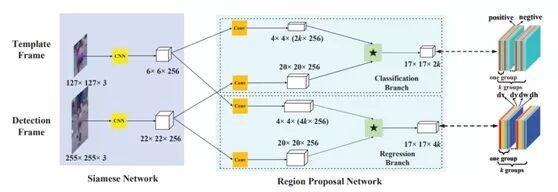SiamRPN improves SiameseFC by introducing region proposal network (RPN) to handle scale changes during the visual object tracking. The response of template and input are sent to the classification branch and regression branch of RPN. In each of the branch, k anchors generated and the cross-correlation are applied between the template and input based on each of the anchor.

# SiamRPN++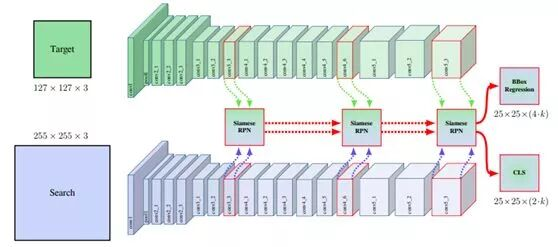SiamRPN++ addresses two limitation of SiamRPN:

• SiamRPN performances degrade with modern deeper backbone, e.g., ResNet, which is caused by padding;
• The classification score and regression score is not longer symmetric as the cross-correlation in SiameseFC.